# Equality of two equations for acoustic resonance of a sphere

#### drewpear

##### New member
howdy and thank you.
for many years i've had an equation to determine the diameter of a sphere to resonate a given frequency.
actually i've had two. one for a sphere with a sound hole and one for a sphere with a hollow neck/tube attached
as the sound hole. both types are used on traditional african xylophones. i would like to check the accuracy of both,
however this post concerns the equation for a sphere with just a sound hole.
i see in wikipedia that the equation, solved for the frequency is -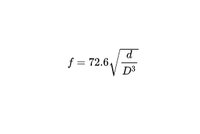my equation is solved for the diameter and it is -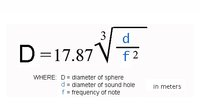is my equation correct according to wikipedia?

thank you,
drew

thank you

#### drewpear

##### New member
hello again.

i would love some help with another equation.
i have this equation to determine the diameter of a sphere with a neck to resonate a given frequency along with other given variables.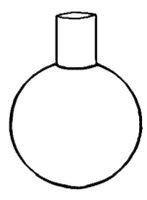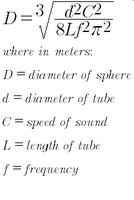i'm hoping to verify it's accuracy using this equation i found on the web.
which i believe may describe the same evaluation (or whatever it may be called)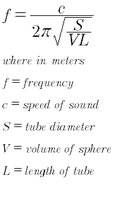my equation came to me a long time ago with assistance and i am unable
to move equations around at this time.

thank you!